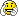# Strange output of TMatrixDEigen::GetEigenVectors()

Hello,

I am trying to work with the matrix classes of ROOT
and i am looking at a simple example:
I have a 3x3 matrix A:

1 2 0
0 3 0
2 -4 2
and I am searching for the eigenvector matrix P which should look like (by hand):
1 0 1
1 0 0
-2 1 -2

Below are the operation I have done with CINT:

``````root  TMatrixD A(3,3)
Error: Can't call @@ ex autload entry remove by typedef declaration @@TMatrixD::@@ ex autload entry remove by typedef declaration @@TMatrixD(3,3) in current scope (tmpfile):1:
Possible candidates are...
*** Interpreter error recovered ***
root  TMatrixD A(3,3)
root  A=1
(const int)1
root  A=2
(const int)2
root  A=2
(const int)2
root  A=3
(const int)3
root  A=-4
(const int)(-4)
root  A=2
(const int)2
root  TMatrixDEigen M(A)
root  TMatrixD P(3,3)
root  P=M.GetEigenVectors()
(class TMatrixT<double>)158895384
root  for(int i=0;i<3;i++) {for(int j=0;j<3;j++) cout<<P[i][j]<<"        "; cout<<endl;}
1        4.44089e-16        -0.447214
1        0        0
-2        -2.23607        0.894427``````

First I get an error that i don t understand.
And my second problem is the output of the second column of the matrix P.
Instead of 0 i get 4.44089e-16. Ok it is still a small number which probably comes from the numerical method. But is there a way to improve this and have directly 0?

thank you for your help

Hi,

[quote]root  TMatrixD A(3,3)
Error: Can’t call @@ ex autload entry remove by typedef declaration @@TMatrixD::@@ ex autload entry remove by typedef declaration @@TMatrixD(3,3) in current scope (tmpfile):1:
Possible candidates are…
*** Interpreter error recovered ***[/quote]is an issue with the ROOT autoloader and CINT which will be solved in the context of ROOT 6 (with Cling).

Cheers,
Philippe.

rootuser01,

Which platform ?? which operating system ??

Neither 5.26/00 on 32-bit Linux or 5.32/00 64-bit MacOSX show either issue.

Your 4e-16 number is 4 times the smallest significand in a double representation so not bad at allThe algorithms used are from EISPACK and I am not aware of any improvements on them.
Just out of curiosity could you run the following little scripts in the ROOT interpreter

``````void eps()
{
// Find eps, the largest double so that 1+eps =1
Double_t eps = .5;
for (Int_t i = 0; i < 10000; ++i) {
eps *= .5;
const Double_t epsp1 = eps+1;
if (epsp1 <= 1 ) break;
}
std::cout << "eps = " << eps << std::endl;
}``````

The result should be eps = 1.11022e-16

Eddy

I can confirm that observation (Ubuntu 10.04.4 LTS, i686, gcc 4.4.3). Neither ROOT 5.28 nor 5.30 have this problem while ROOT 5.34 breaks (I mean “TMatrixD A(3,3) Error: Can’t call @@ ex autload entry …”).
In any case, I get: ```1 0 -0.447214 1 0 0 -2 -2.23607 0.894427 eps = 1.11022e-16```